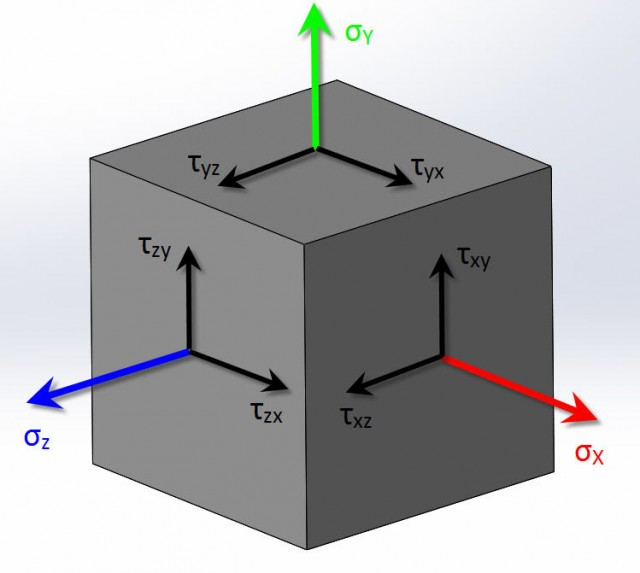# Where are the other Shear Stress Simulation Plots?Article by Scott Durksen, CSWE updated November 19, 2014

###### Article

Have you ever needed a shear stress plot from your study but didn’t find the right direction and plane?  When creating shear stress plots in SOLIDWORKS Simulation, there are only three options.  But where are the other three shear stresses?

Well due to the equilibrium requirement of the elementary cube (not the FEA tetrahedral element), there are three sets of equal shear stresses.  On the elementary cube, the shear stresses that are pointing to each other on perpendicular sides shared with an edge will be equal.   Therefore the shear stress plots of the other orientations would have the exact same values as the three that are available.

Due to the equilibrium:

TXY = TYX

TXZ = TZX

TYZ = TZYI have a hard time remembering the directions and planes based on the symbols (i.e. TYX), so here are the equalities in word form:

(TXY) Shear in Y Dir on YZ Plane = (TYX) Shear in X Dir on XZ Plane

(TXZ) Shear in Z Dir on YZ Plane = (TZX) Shear in X Dir on XY Plane

(TYZ) Shear in Z Dir on XZ Plane = (TZY) Shear in Y Dir on XY Plane

#### Get Certified SOLIDWORKS Services from Javelin